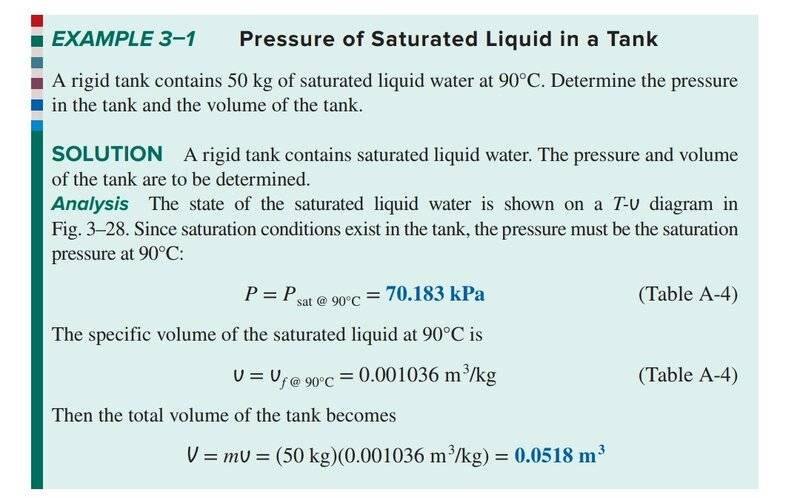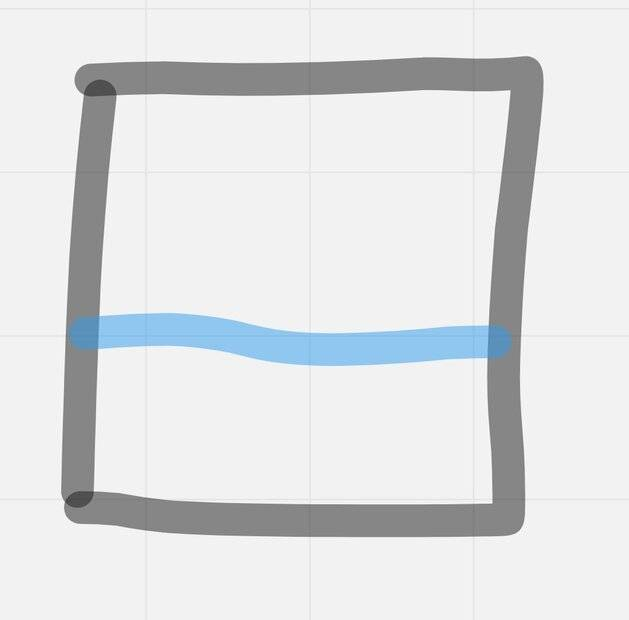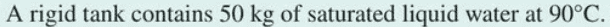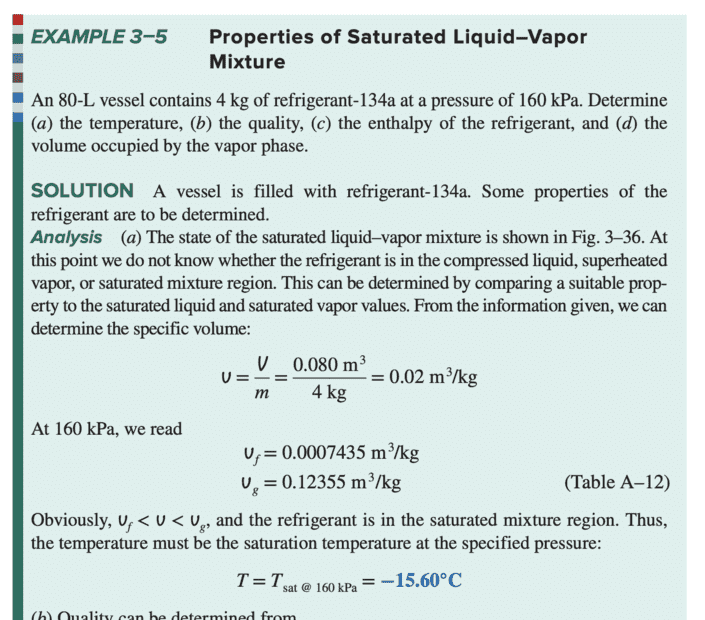# Confusion about the interpretation of specific volume

• I
EddiePhys
So I'm reading through Cengel's thermodynamics textbook, and came across this solved example:Firstly, pressure in this context I'm assuming is vapour pressure? Since we're dealing with pure substances in this chapter.

But what's confusing me is, here's the diagram I have:They've not mentioned that the water fills the tank. So I don't understand why the specific volume times the mass of the water equals the volume of the tank?

They've used similar reasoning in following solved examples as well where it's unclear that the liquid will occupy the tank volume

Last edited:

Homework Helper
They've not mentioned that the water fills the tank.I think you can safely take it that there is nothing else present, i.e. no water vapour...

##\ ##

•russ_watters and Chestermiller
EddiePhys
View attachment 320034
I think you can safely take it that there is nothing else present, i.e. no water vapour...

##\ ##

They have used similar lines of reasoning in future solved examples where it's not so clear that the tank will be filled with liquid

For example, here, when they compare the ##v## value with ##v_g## and ##v_f##, to determine the state, it would only make sense if, when it is in liquid state, it is occupying the entire vessel:Mentor
They have used similar lines of reasoning in future solved examples where it's not so clear that the tank will be filled with liquid

For example, here, when they compare the ##v## value with ##v_g## and ##v_f##, to determine the state, it would only make sense if, when it is in liquid state, it is occupying the entire vessel:

View attachment 320044
I guess you weren't satisfied with my answers in that other forum.

Homework Helper
Yes, there is a subtle difference between "4 kg of refrigerant" and "50 kg of saturated liquid water" .
You'd better learn to distinguish the difference in these simple exercises -- practice for later when it's not so easy any more.##\ ##

•russ_watters
EddiePhys
I guess you weren't satisfied with my answers in that other forum.

I really appreciated your answers. Something just wasn't clicking with me. So I thought getting different perspectives would perhaps help. Please don't take it personally, I really do appreciate your help.

Mentor
The largest volume that 4 kg of refrigerant 134a can occupy as a liquid at 160 kPa is (0.0007435)(4)=0.0030 m^3 = 3.0 liters

EddiePhys
The largest volume that 4 kg of refrigerant 134a can occupy as a liquid at 160 kPa is (0.0007435)(4)=0.0030 m^3 = 3.0 liters

Yes. So what I'm not getting is why they're dividing the volume of the vessel by 4kg. They're then comparing this value with 0.0007435. The two would only be equal if they were the volume of the liquid by 4kg (or equivalently, that the vessel was fully filled, in which case dividing the volume of the vessel by 4kg is the same as dividing the volume of the liquid).

So in the case that the liquid only occupies a small portion of the volume of the vessel, volume of the vessel divided by 4kg would not be suitable means to assess if it's in compressed liquid state

Mentor
Yes. So what I'm not getting is why they're dividing the volume of the vessel by 4kg. They're then comparing this value with 0.0007435. The two would only be equal if they were the volume of the liquid by 4kg (or equivalently, that the vessel was fully filled, in which case dividing the volume of the vessel by 4kg is the same as dividing the volume of the liquid).
The mass of refrigerant is way less than sufficient to fill the tank with liquid alone.. So there must be mixture of liquid and vapor in the tank. At equilibrium, we must have that : $$m_Lv_L+m_Vv_V=V$$Doo you agree with this?
So in the case that the liquid only occupies a small portion of the volume of the vessel, volume of the vessel divided by 4kg would not be suitable means to assess if it's in compressed liquid state
This would mean that it can't be in a state of compressed liquid (i.e., liquid at a pressure greater than the vapor pressure at the tank temperature).

EddiePhys
The mass of refrigerant is way less than sufficient to fill the tank with liquid alone.. So there must be mixture of liquid and vapor in the tank. At equilibrium, we must have that : $$m_Lv_L+m_Vv_V=V$$Doo you agree with this?

This would mean that it can't be in a state of compressed liquid (i.e., liquid at a pressure greater than the vapor pressure at the tank temperature).

Yes, agreed with the first part. And this would hold even for the compressed liquid state, correct?

The second part, what I'm thinking is: imagine a piston pushing down on a liquid contained in a cylinder with some pressure. This compresses the liquid. So wouldn't the the specific volume for a given pressure be equal to the volume of this liquid divided by the mass. And this would be the value reported in tables.

In our case we're dividing the volume of an arbitrary vessel by the mass of the liquid and comparing it to the above value.

Mentor
Yes, agreed with the first part. And this would hold even for the compressed liquid state, correct?

The second part, what I'm thinking is: imagine a piston pushing down on a liquid contained in a cylinder with some pressure. This compresses the liquid. So wouldn't the the specific volume for a given pressure be equal to the volume of this liquid divided by the mass. And this would be the value reported in tables.
Yes, reported in tables for compressed liquid.
In our case we're dividing the volume of an arbitrary vessel by the mass of the liquid and comparing it to the above value.
The value we calculate is between that of a saturated liquid at 160 kPa and a saturated vapor at 160 kPa. So we must have a combination of the two.

EddiePhys
Yes, reported in tables for compressed liquid.

The value we calculate is between that of a saturated liquid at 160 kPa and a saturated vapor at 160 kPa. So we must have a combination of the two.

But even if it was a compressed liquid, we wouldn't know because we're not dividing the volume of the liquid by its mass, and this is the value that is given in tables and that we're using as a standard for comparison.

Mentor
But even if it was a compressed liquid, we wouldn't know because we're not dividing the volume of the liquid by its mass, and this is the value that is given in tables and that we're using as a standard for comparison.
If it was a compressed liquid, the tank volume divided by the msss of refrigerant in tank would be less than the specific volume of the saturated liquid.

EddiePhys
If it was a compressed liquid, the tank volume divided by the msss of refrigerant in tank would be less than the specific volume of the saturated liquid.

Why though. Let's say V1 is the volume of the compressed liquid and V2 the tank. And V2>>V1. In this case, the specific volume of the compressed liquid would be calculated V1/M in the tables while a saturated liquid at that pressure might have a volume in the vicinity of V1, let's say V1 plus a since liquids aren't that compressible. But we're calculating it as V2/M and V2>>V1, so it why shouldn't the tank volume give the larger answer

Mentor
Why though. Let's say V1 is the volume of the compressed liquid and V2 the tank. And V2>>V1. In this case, the specific volume of the compressed liquid would be calculated V1/M in the tables while a saturated liquid at that pressure might have a volume in the vicinity of V1, let's say V1 plus a since liquids aren't that compressible. But we're calculating it as V2/M and V2>>V1, so it why shouldn't the tank volume give the larger answer
If it is "compressed liquid," then there is no vapor in the tank, and ##V_1=V_2##. In this case, $$\frac{V_1}{M}=\frac{V_2}{M}<v_L(T)$$where ##v_L(T)## is the specific volume of saturated liquid at the tank contents temperature T. So the specific volume is a little less than the specific volume of the saturated liquid, and the tank is filled with liquid if the pressure is above the saturation vapor pressure at T.

EddiePhys
If it is "compressed liquid," then there is no vapor in the tank, and ##V_1=V_2##. In this case, $$\frac{V_1}{M}=\frac{V_2}{M}<v_L(T)$$where ##v_L(T)## is the specific volume of saturated liquid at the tank contents temperature T. So the specific volume is a little less than the specific volume of the saturated liquid, and the tank is filled with liquid if the pressure is above the saturation vapor pressure at T.

Okay I think now I am close to getting it. What would the case then be where V1 << V2 AND there's no vapor in the tank? Or is this a case we simply don't consider. So we only have 1) tank full of liquid,2) liquid + gas, 3) only gas as possibilities. And if I understand correctly, saturated liquid is almost like (1) i.e fully filled tank but slightly larger volume

Last edited:
Mentor
Okay I think now I am close to getting it. What would the case then be where V1 << V2 AND there's no vapor in the tank? Or is this a case we simply don't consider.
If there is no vapor in the tank, then ##V_1=V_2##
So we only have 1) tank full of liquid,2) liquid + gas, 3) only gas as possibilities.
Yes.
And if I understand correctly, saturated liquid is almost like (1) but a tiny portion is gas
Purely saturated liquid is like (1) but with essentially no vapor.

EddiePhys
If there is no vapor in the tank, then ##V_1=V_2##

Yes.

Purely saturated liquid is like (1) but with essentially no vapor.

"If there's no vapor in the tank, then ##V_1=V_2##" So you can't simply have a liquid with a volume less than the tank volume. The second you do, you are in the region to the right of the saturated liquid phase (i.e saturated liquid-gas mixture)

•Chestermiller
EddiePhys
I'm taking you liking the post to mean yes. In which case, I think I got it! Head's a bit hazy right now so I'll know for sure by tomorrow morning, but thank you so much!! I really appreciate you taking the time you did to answer my questions